# Procedures for Testing the Significance of a Linear Model using ETM

Ron Eastman

A test of significance for a linear model applies equally to the slope of the regression and the correlation coefficient. However, the presence of serial correlation in the residuals from the model is a major issue that may make this test invalid. This is common and needs special attention before a test of significance can be evaluated. To illustrate the problem, consider a model that tries to relate lower tropospheric temperature anomalies (TLTA) in Venezuela to the Oceanic Nino Index (ONI) – an index to the ENSO phenomenon. Here is a graph that aggregates all of Venezuela for TLTA (Y) versus the ONI (X):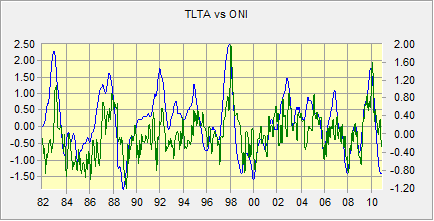Figure 1 : The ONI index series (in blue) and a profile of TLTA for Venezuela (in green).

The correlation is quite high (r = 0.54), but is it significant? Now let’s look at the residuals. ETM allows you the option of creating a residual series when you compute a linear model. Here is a temporal profile using the boundary of Venezuela to define the sample: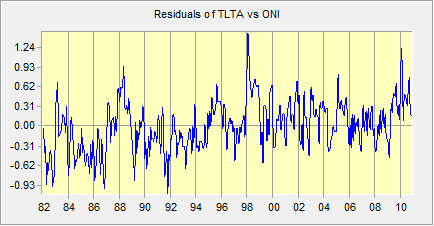Figure 2 : A profile of the residuals of the relationship between ONI (X) and TLTA (Y) for Venezuela.

The lag 1 serial correlation in this series is rs = 0.65, which is quite high. Anything greater than 0.05 should be accommodated in the procedures used to test for significance (Wang and Swail, 2001).

### Impact of Serial Correlation

So what is the impact of serial correlation in the residuals? Serial correlation in the residuals has no impact on the linear model correlation, r – it is unbiased. However, estimates of statistical significance may be exaggerated because the estimated variance of r will tend to be too small. Thus you are very susceptible to reporting a significant relationship when it is not warranted.

### Causes of Serial Correlation

There are many possible causes of serial correlation, including (Kirchner, 2013):

1. Model specification error. Are there any missing explanatory variables? Although you may be interested in the relationship with one variable, it is important to include as many major sources of variation as possible (bearing in mind the basic tenet of parsimony).
2. Long-term trend. This does appear to be the case with the illustration above.
3. Many earth science data exhibit inertia. The oceans, for example, cannot instantly change temperature in response to a temperature change. Another example is vegetation productivity, such as is measured by NDVI. If the rains suddenly stop, the vegetation takes time to respond since the soil contains adequate moisture to maintain the plants for a period of time.

### Coping with Serial Correlation

There are several possible ways of handling serial correlation. This discussion is oriented specifically to earth science problems typically analyzed using the linear modeling procedure in ETM.

1. Focus first on incorporating missing variables. As you build up your model, assess the serial correlation in the residuals at each stage (as will be discussed below). You should see the serial correlation decline with the addition of each meaningful independent variable.
2. Accommodate trend. If the residuals still exhibit a trend, incorporate time as an independent variable. In ETM, the easiest way to do this is to create a linear index series using the Generate/Edit Series panel of the preprocess tab. Add the resulting series as an additional independent variable.
3. After you have exhausted your ability to improve the specification of the model, calculate the serial correlation of the residuals (as will be discussed below), rs, and then adjust the effective sample size to accommodate the remaining serial correlation, where the effective sample size ne is (Nychka et al., 2000):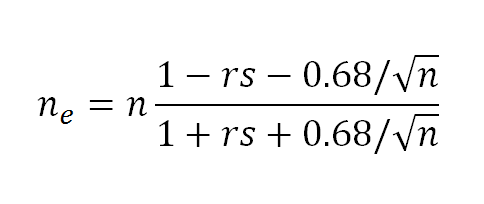Note that if ne < 6, then there is an inadequate effective sample size to estimate model significance.

1. Calculate Student’s t for the model using the following formula (PSU, 2013):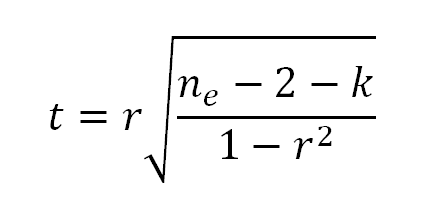with ne-2-k degrees of freedom, where k is the number of variables upon which the relationship is conditioned. The variable k applies only in the case of partial correlation. If the correlation is not a partial correlation, k =0. However, when testing a partial correlation, k is the number of variables held constant.

Note that using ETM, the linear model is calculated independently at each pixel. Therefore these calculations are done with images. Either Image Calculator or MacroModeler will facilitate this process, as will be illustrated below.

### Other Approaches

There are other approaches to handing serial correlation. However, there are issues that are of concern.

1. The first alternative would be to repeat the steps above, but instead of calculating an effective sample size in Step 3, the series is subsampled to a coarser time step until the serial correlation disappears. This works fine, but we feel there is an advantage to estimating linear model parameters (which remain unbiased) using all of the data. Also, with the suggested procedure above involving a calculation of ne, no decision is required about what new sampling interval to use.
2. The second alternative is to use one of the family of procedures that transforms the data to remove the serial correlation. These include the techniques of first lag differencing, the Cochrane-Orcutt transformation and Trend Preserving Prewhitening, all offered by ETM. For this type of application, we don’t recommend these. Applying one of these transformations is VERY sensitive to the original temporal sampling interval. If the original temporal sampling interval is short relative to the interval required for independence, there is a strong likelihood that the procedure will discard the signal along with the noise (Cochrane, 2012). For example, in the case study here, if we difference both the ONI and the TLTA data then the relationship being evaluated is not between the sea surface temperature in the central Pacific and atmospheric temperature, but rather, between changes in ONI versus changes in TLTA over the sampling interval. If the sampling interval is fine (e.g., weekly), then we would expect that the changes in ONI and TLTA would be very small, and perhaps dominated by noise and measurement error rather than the influence of ENSO. We might then conclude that there is no relationship when in fact there is (i.e., we just threw the relationship away). Note, however, that if the temporal sampling interval is chosen appropriately, these techniques work fine.

## Procedural Notes

### Step 1: Model Development

In the model development stage, we suggest you evaluate the degree of serial correlation in the residuals at each stage. This can be done by asking for a residual series along with your output (there is a checkbox for this). Then assess the serial correlation in your residual series by running a Durbin-Watson test (one of the options in the Detrend/Prewhiten panel of the Preprocess tab). Values around 2 indicate no serial correlation, while values below or above 2 indicate positive or negative autocorrelation, respectively. You can use a standard statistics text to determine a critical threshold for significant autocorrelation based on your series length. Be concerned only with the serial correlation in your specific study area and ignore areas outside. You want to get the serial correlation as low as possible by adding explanatory variables, but do not keep an explanatory variable if it is not making an appreciable difference.

To help with your search for explanatory variables, try creating a temporal profile of the residuals in your study area. Then save the profile as an index series. Then use linear modeling to see what areas are related to the residual profile. For example, a linear model was run between the ONI index (as the independent variable) and the TLTA series. Here was the resulting correlation image: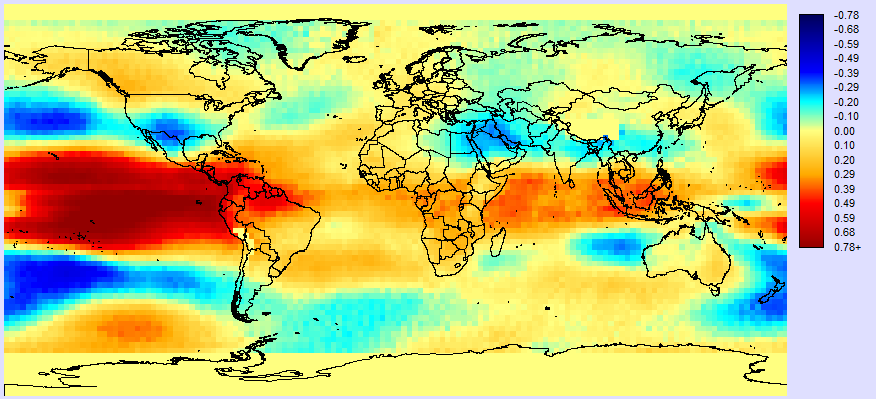Figure 3 : The correlation image between the ONI index (X) and TLTA (Y).

In addition, a residual series was created when this model was run. The mean Durbin-Watson statistic in the residual series for Venezuela is 0.95, which is a 30% improvement over the Durbin-Watson statistic for the raw TLTA data for this region. A profile of the residual series was then created using the Explore Temporal Profiles panel in the Explore tab. The map of country boundaries was used to define the profile region as Venezuela. The result was the profile in Figure 2.

The question now is, does the profile of the residual suggest a variable that might be missing? There is a strong trend. Could it be global warming? Could it be some other ocean climate teleconnection? Ocean teleconnections (such as ENSO) are a major potential source of variability so let’s save the profile as an index series and run a linear model using the profile as the X variable and sea surface temperature anomalies (SSTA) as the dependent variable. Here is the result: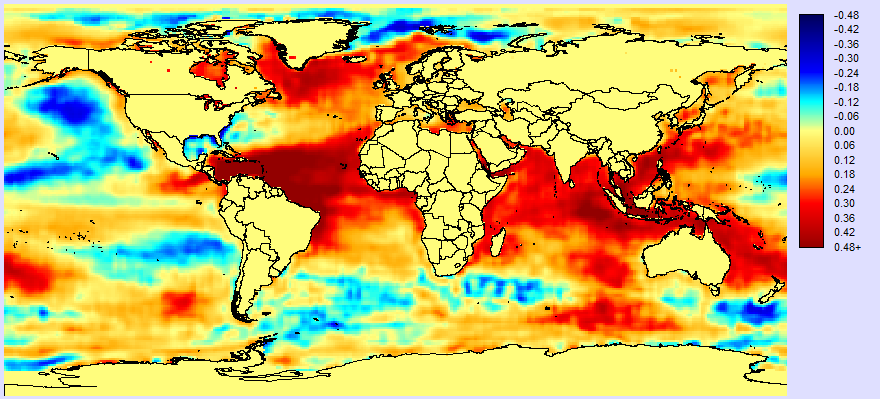Figure 4 : Correlation between the residual profile (in Fig. 2) and SSTA.

The result looks remarkably like some mix between the Atlantic Multidecadal Oscillation (AMO) and possibly global warming. To confirm this, here is a graph of the residual profile and the published AMO index (from NOAA):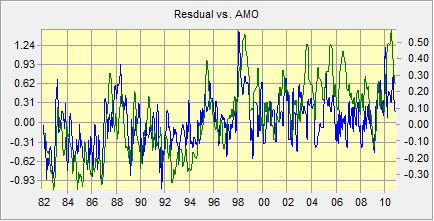Figure 5 : The residual profile (in blue) and the AMO index (in green). The correlation between them is 0.57.

The correlation between these two is high: +0.57. In addition, it can be seem that the AMO index has both the long term trend and much of the shorter term variability. Therefore the AMO needs to be added as a variable.

After adding the AMO as the second explanatory variable (along with the ONI), the multiple correlation between TLTA and the ONI and AMO looks as follows: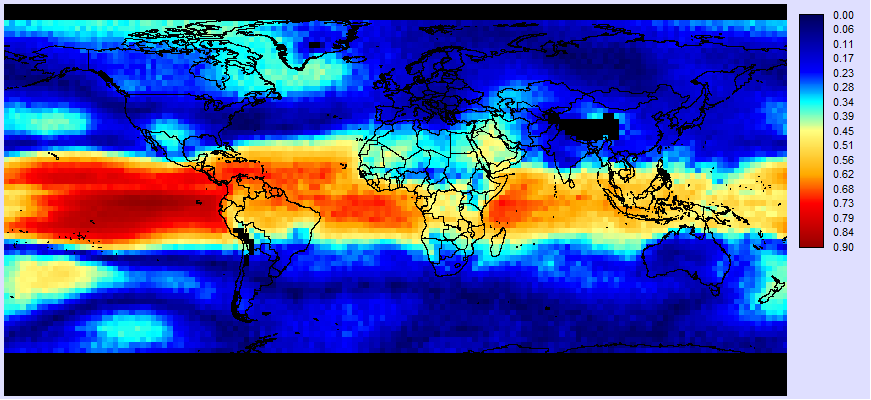Figure 6 : The multiple correlation between TLTA (Y) and ONI (X1) and AMO (X2).

The correlations in Venezuela are quite high – characteristically around +0.65. Meanwhile, the residual profile in Venezuela looks like this:Figure 7 : Profile of the residuals in Venezuela from a model between the ONI (X1), AMO (X2) and TLTA (Y).

The pattern is now looking fairly incoherent, but still has some serial correlation. The mean Durbin-Watson statistic for Venezuela is 1.26. This is 33% improvement over just using the ONI alone. However, it still indicates that there is serial correlation. A statistics text indicates that values lower than 1.653 for this large a sample (348 months) and two regressors should be considered as positively serially correlated at the 1% probability level. Since there isn’t much in the way of a coherent temporal pattern, perhaps this remaining serial correlation relates to inertia. Let’s therefore decide that our model is complete (as best we can determine) and move to the next stage.

### Step 2: Estimate the Degree of Serial Correlation and the Effective Sample Size

In the previous step we used summary values for the whole study area in refining our model. However, at this stage we will work at the pixel level within the study area. The first thing we need is an estimate for the degree of serial correlation (rs). To do this, we will use the linear modeling tool again. First, indicate that the independent variable will be an image series and select the residual series for the full model. Then select the same series as the dependent variable. Then, in the column marked lag for the independent series, specify 1. Then select R as the output and run the model. Here is the result: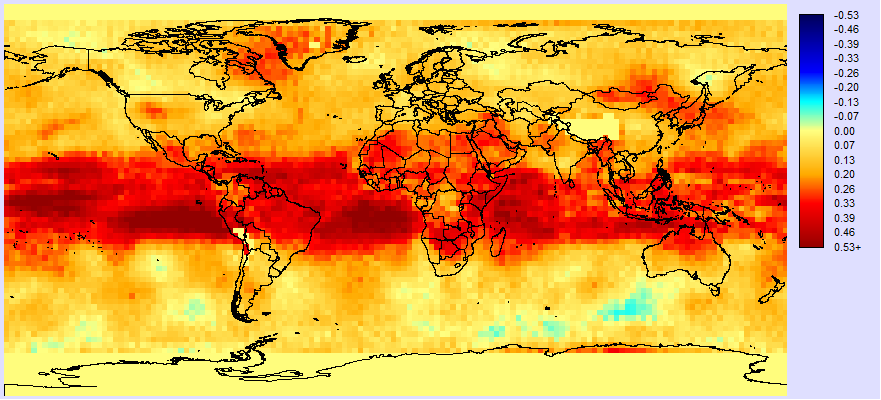Figure 8 : Estimated serial correlation (rs) of the residuals from the full model: TLTA = f(ONI,AMO).

The pattern is interesting. The highest correlations are in the tropics. There is an interesting atmospheric bridge phenomenon whereby tropical ocean warming leads to convective warming in the atmosphere which is subsequently propagated across the tropics. This may account for much of the remaining serial correlation in our model – a kind of inertia effect. In the Venezuela area, the estimated serial correlation varies from pixel to pixel, but is typically around +0.37.

The next step is to use Image Calculator to calculate the effective sample size using the formula above. Here is a screen shot for this case study: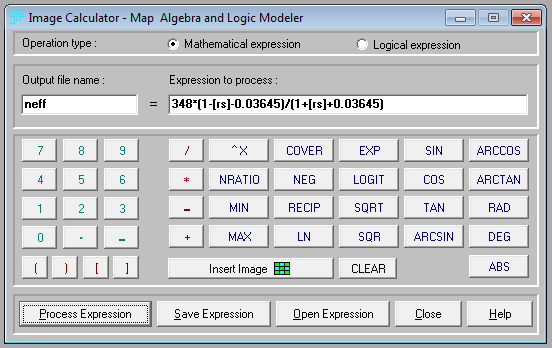Figure 9

[rs] is the name of estimated serial correlation image in Figure 8 (using the Image Calculator convention of square brackets to indicate images). 348 is the original sample size (n). 0.03645 is a reduction of 0.68/sqrt(n). In Venezuela, the effective n is typically around 148. Figure 10 shows the image of ne determined by the formula in Figure 9.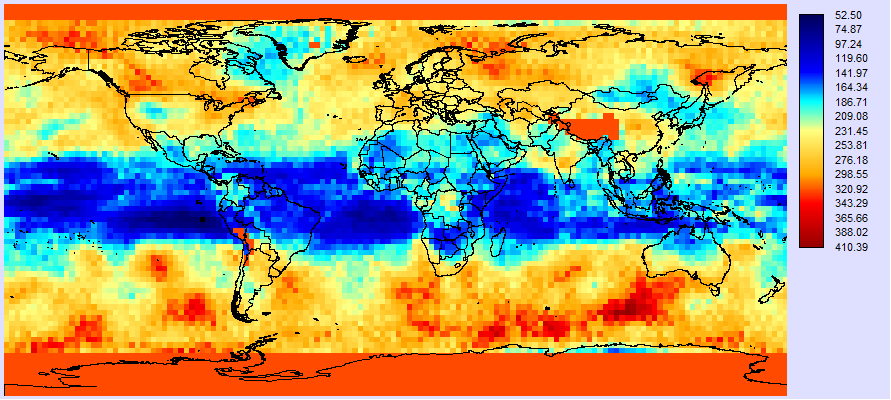Figure 10 : The image of effective sample size (ne).

### Step 3: Determining the significance of the Correlations

We are now ready to do the final step – to calculate the t-score for the significance of the correlations. If we are testing the full model, k in the equation above is 0, and thus the degrees of freedom are ne-2. Figure 11 shows the Image Calculator setup where [tlt_oni_amo_lm_r] is the multiple correlation for the full model using the ONI and AMO as independent variables, while Figure 12 shows the result.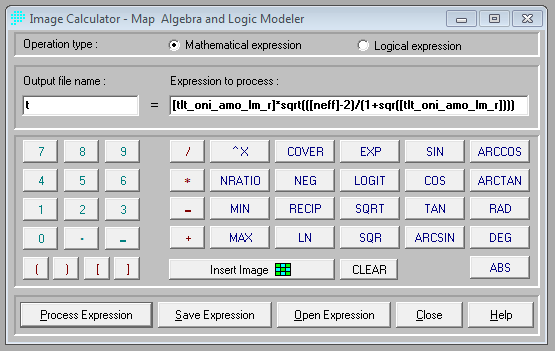Figure 11

As can be seen in Figure 12, the t values in Venezuela are quite high – typically around 6.56. With the degrees of freedom typically around 148-2=146 for Venezuela, t is approaching z and we can safely conclude that the correlation is significantly different from 0 at the p<0.001 level throughout Venezuela.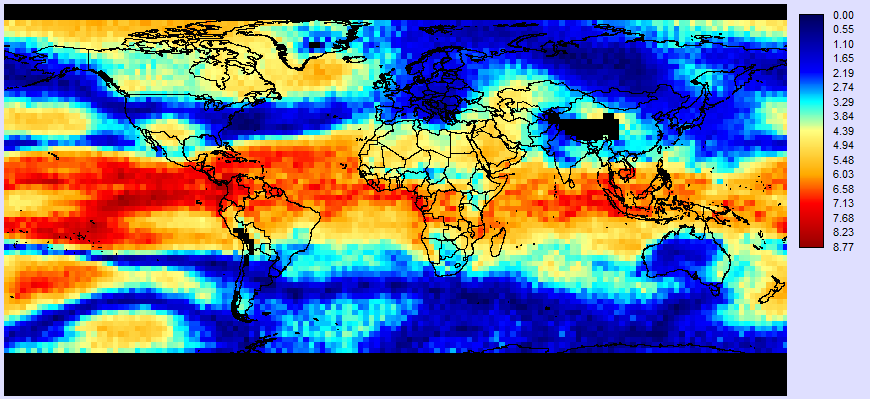Figure 12 : The value of t for the regression of TLTA (Y) versus ONI (X1) and AMO (T2).

Note that if you wish to test one of the partial regressions, simply modify the numerator of the equation to calculate t to include the variable k. In this example, if we were testing the partial correlation of the ONI while holding the AMO constant, k would equal 1 and the degrees of freedom would be ne-2-k.

## References

Cochrane, J.H., (2012) A Brief Parable of Over-Differencing, (unpublished manuscript) http://faculty.chicagobooth.edu/john.cochrane/research/papers/overdifferencing.pdf

Kirchner, J.W. (2013) http://seismo.berkeley.edu/~kirchner/eps_120/Toolkits/Toolkit_11.pdf, accessed Aug 18, 2013.

Nychka, D., Buchberger, R., Wigley, T.M.L., Santer, B.D., Taylor, K.E., Jones, R.H., (2000) Confidence intervals for trend estimates with autocorrelated observations, (unpublished manuscript) http://citeseerx.ist.psu.edu/viewdoc/download?doi=10.1.1.33.6828&rep=rep1&type=pdf

PSU, (2013) http://sites.stat.psu.edu/~ajw13/stat505/fa06/08_partcor/06_partcor_partial.html, accessed Aug. 18, 2013.

Wang, X.L., and V.R. Swail, 2001. Changes of extreme wave heights in northern hemisphere oceans and related atmospheric circulation regimes, Journal of Climate, 14, 2204-2221.

 The terms serial correlation and autocorrelation are synonymous.

 To calculate the lag correlation of a profile, save it as an index series. Then display the index series using the Explore panel. Next, add the same index as a second series. Now lag it by 1 month and look at the correlation.

 rs will be used to indicate the first lag serial correlation coefficient to distinguish it from the linear model correlation, r.

 The warming in the southern Caribbean and in the Labrador Sea suggest this, in particular.